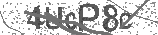﻿ Circles - Area, Circumference, Radius, & Diameter Explained! | Safe Videos for Kids
Welcome

# Circles - Area, Circumference, Radius, & Diameter Explained!

Thanks! Share it with your friends!

URL

You disliked this video. Thanks for the feedback!

Sorry, only registred users can create playlists.URLChannel: The Organic Chemistry Tutor
Categories: Geometry   |   MathFind Related Videos  added
14 Views## Description

This basic geometry video tutorial explains how to calculate the area and circumference of a circle given it's radius and diameter. This video contains a few examples with word problems. This video also explains how to calculate the circumference given the area, and how to calculate the length of the radius and diameter given the area and circumference of the circle. This video contains plenty of examples and practice problems.

Pre-Algebra Video Playlist:Be the first to comment